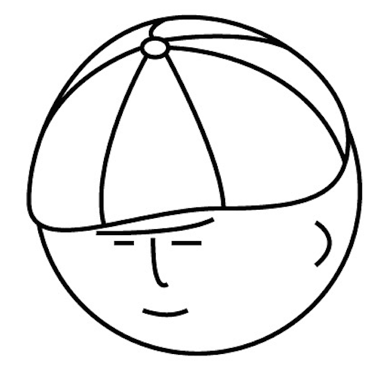マツシタのお勉強メモ

マツシタのお勉強

welcome to my engineering blog
Hacker RankImplement a Queue by using two Stacks

Problem Implement a MyQueue class which implements a queue using two stacks Source Code

Towers of the Hanoi in Java

Problem In the classic problem of the Towers of Hanoi, you have 3 towers and N discs of different sizes which can slide onto any tower. How to Solve First we think about sliding nth disc onto another tower. This handling is composed by the…

Implement SetOfStacks

Problem Implement a data structure SetOfStacks. SetOfStacks should be composed of several stacks and should create a new stack once the previous one exceeds capacity. SetOfStacks.push() and .pop() should behave identically to a single stac…

Implement function min which returns minimum element in stack

Problem How would you design a stack which, in addition to push and pop, also has a function min which return minimum element? Push, pop and min should all operate in O(1) time. How to Solve By keeping a minimum value at each state, we can…

Use a single array to implement three stacks

Problem Describe how you could use a single array to implement three stacks. We can divide the array in three equal parts. So we use the array like this. For stack 1, we will use [0, n/3) For stack 2, we will use [n/3, 2n/3) For stack 3, w…

Implementation of Stack with LinkedList in Java

Implementation of Stack with LinkedList I defined the Stack class with a top property which is defined as the following explanation. top: the position to insert next Node at next time and the position to get a Node at next time. When I pus…

Longest Absolute File Path - Past Goolge Interview

Problem leetcode.com We are given a string as a file path like this: "dir\n\tsubdir1\n\tsubdir2\n\t\tfile.ext". This string means directory structure below. We answer a number of max length of file path like this: 20 (number of length abou…